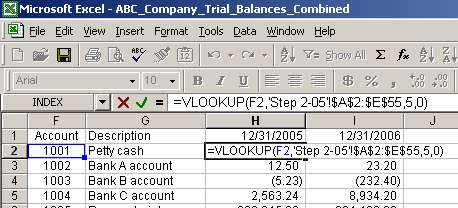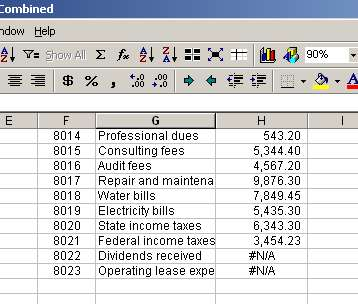# Vlookup and Trial Balances Function and Example

### 4) Pull data from trial balances into combined trial balance file

We have prepared the file structure to build a comparative trial balance. To pull trial balance data from 12/31/05 and 12/31/06 tabs into one tab, we will use a combination of Excel functions IF, ISERROR and VLOOKUP.

First, let us add two captions to the combined trial balance file so that we don't lose track of periods. In cell H1 and I1 we enter 12/31/2005 and 12/31/2006 respectively (in the file, see tab Step 4):Second, we will use function VLOOKUP to pull data from 12/31/05 and 12/31/06 trial balances. Let us start with 12/31/05. In cell H2, we enter the following formula:

= VLOOKUP(F2,'Step 2-05'!\$A\$2:\$E\$55,5,0)

You can see, that argument "F2" is a reference to the account number for which we want to pull the balance. "'Step 2-05'!\$A\$2:\$E\$55" is the range on tab "Step 2-05" which contains the trial balance for 12/31/05. Argument "5" is the 5th column in the above named range ("Debit / Credit"). And finally the last argument is 0 (you can read more about this argument in the Excel help file to get more information).

The result is 20.30. We then copy the formula to the end of the range in column H:After pasting the VLOOKUP formula in column H, scroll down to rows 56 and 57. You will notice that cells H56 and H57 have "#N/A" in them:Page 5 of 7